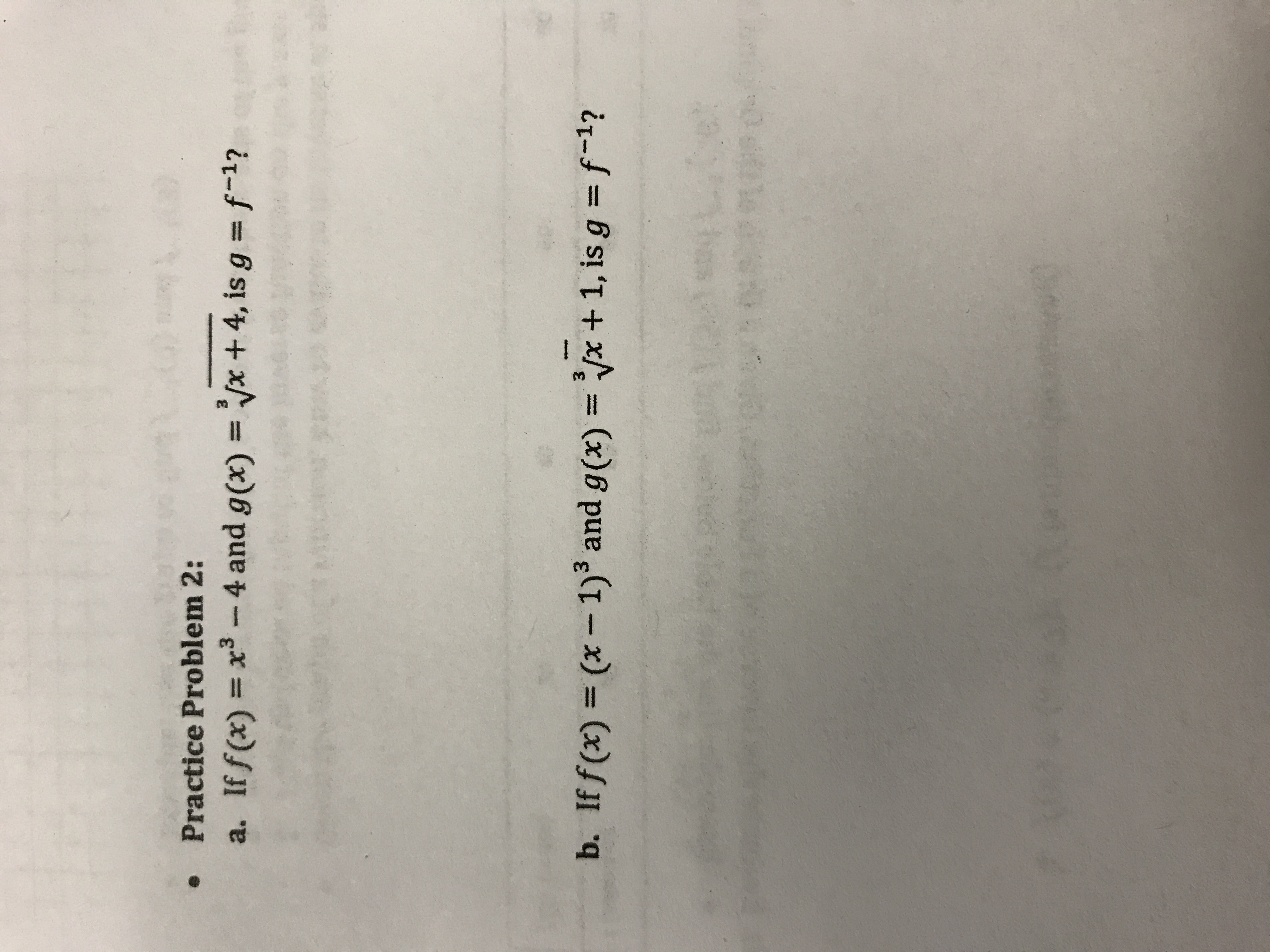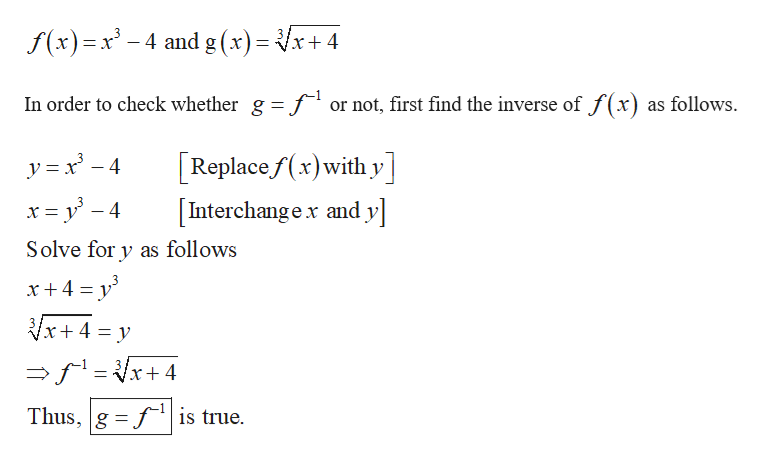# Practice Problem 2:3a. If f(x)x3 - 4 and g(x)=x + 4, is g f-1?b. If f (x) (x -1)3 and g(x)x + 1, is g f~-1?10

Question
6 views

step by step to see if each problem can inverse of each otherhelp_outlineImage TranscriptionclosePractice Problem 2: 3 a. If f(x) x3 - 4 and g(x)=x + 4, is g f-1? b. If f (x) (x -1)3 and g(x)x + 1, is g f~-1? 10 fullscreen
check_circle

Step 1

a. The given function...help_outlineImage Transcriptionclosex+4 f(x)x-4 and g(x)=- In order to check whether g = for not, first find the inverse of f(x) as follows Replacef(x)with y yx-4 Interchangex and y х- Solve for y as follows x+4 y Vx+ 4 y =x4 Thus, g fis true. fullscreen

### Want to see the full answer?

See Solution

#### Want to see this answer and more?

Solutions are written by subject experts who are available 24/7. Questions are typically answered within 1 hour.*

See Solution
*Response times may vary by subject and question.
Tagged in

### Calculus